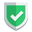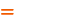我们相信：世界是美好的，你是我也是。平行空间的世界里面，不同版本的生活也在继续...

## 代码对比

``````a = b = "init"
b = "modify"
print(a,b)``````

``init modify``

``````import pandas as pd
from pandas import Series, DataFrame
df = DataFrame([
('虎子', 5, "puppy"),
('老许', 3, "catty"),
],
columns=('name', 'age', 'class')
)
df2 = df
df.pop("age")
print(df)
print(df2)``````

``````  name class
0   虎子   puppy
1   老许   catty
name class
0   虎子   puppy
1   老许   catty``````

## 正确的操作方式

``````import pandas as pd
from pandas import Series, DataFrame
df = DataFrame([
('虎子', 5, "puppy"),
('老许', 3, "catty"),
],
columns=('name', 'age', 'class')
)
df3 = df.copy()
df.pop("age")
print(df)
print(df3)``````

``````  name class
0   虎子   puppy
1   老许   catty
name  age class
0   虎子    5   puppy
1   老许    3   catty``````

``df3 = df.copy()``

## 错误的推论

``````b = "init"
a = b.copy()
b = "modify"
print(a,b)``````

``AttributeError: 'str' object has no attribute 'copy'``

## 总结

`pandas``dataframe`对象，不按常理出牌，导致新人掉进陷阱。更多`pandas`的代码使用经验文章，请点击：如果本文对您有帮助，或者节约了您的时间，欢迎打赏瓶饮料，建立下友谊关系。本博客不欢迎：各种镜像采集行为。请尊重原创文章内容，转载请保留作者链接。【福利】 腾讯云最新爆款活动！1核2G云服务器首年50元！【源码】本文代码片段及相关软件，请点此获取更多信息【绝密】秘籍文章入口，仅传授于有缘之人python    pandas